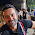VHDL coding tips and tricks: Simple 4 : 1 multiplexer using case statements

## Saturday, March 27, 2010

### Simple 4 : 1 multiplexer using case statements

Here is the code for 4 : 1 MUX using case statements.The module contains 4 single bit input lines and one 2 bit select input.The output is a single bit line.

library IEEE;
use IEEE.STD_LOGIC_1164.ALL;

entity multiplexer4_1 is
port (
i0 : in std_logic;
i1 : in std_logic;
i2 : in std_logic;
i3 : in std_logic;
sel : in std_logic_vector(1 downto 0);
bitout : out std_logic
);
end multiplexer4_1;

architecture Behavioral of multiplexer4_1 is
begin

process(i0,i1,i2,i3,sel)
begin
case sel is
when "00" => bitout <= i0;
when "01" => bitout <= i1;
when "10" => bitout <= i2;
when others => bitout <= i3;
end case;
end process;

end Behavioral;

The testbench code used for testing the code is given below:

LIBRARY ieee;
USE ieee.std_logic_1164.ALL;

ENTITY testbench IS
END testbench;

ARCHITECTURE behavior OF testbench IS
SIGNAL i0,i1,i2,i3,bitout :  std_logic:='0';
SIGNAL sel :  std_logic_vector(1 downto 0):="00";
BEGIN
UUT : entity work.multiplexer4_1 port map(i0,i1,i2,i3,sel,bitout);

tb : PROCESS
BEGIN
i0<='1';
i1<='0';
i2<='1';
i3<='0';
sel <="00";
wait for 2 ns;
sel <="01";
wait for 2 ns;
sel <="10";
wait for 2 ns;
sel <="11";
wait for 2 ns;
--more input combinations can be given here.
END PROCESS tb;

END;

The simulated testbench waveform is shown below:

The code was synthesized using XILINX ISE XST . The RTL schematic of the design is shown below.

Note :- Use RTL Viewer to get a closer look on how your design is implemented in hardware.

#### 8 comments:

1.can you please explain the dataflow model...

2.it's very easy but how I write code for 8 bits multiplexer?
problem is:
a and b 8 bot input, c is 2 bit input , D is 8bit output if c="00" output A, c="01" output B, c="10" output D, c="11" output Z
help me how I write Its code

3.What is the difference between your architecture code and the following one from synthesis point of view?
In case of pure combinational logic (like the described multiplexer) why a process with all the inputs in the sensitivity list should be used instead of the version below?

maybe its the same, or maybe I miss some important difference between the two different descriptions!
thanks!

architecture Behavioral of multiplexer4_1 is
begin

bitout <= i0 when sel="00" else
i1 when sel="01" else
i2 when sel="10" else
i3 ;

end Behavioral;

4.actually your syntext is totally wrong...either use elsif.or use other architecture....

5.programme vhdl d'un multiplexeur 4-1 en utilisant 2 demi multiplexeurs

6.i am a beginner to vhdl..... Can u explain how i can see the rtl schematic in the form of AND , XOR..... i get them as mere boxes with corresponding inputs and outputs........

7.The code was nice

8.Is there any problem if I use signal sel : std_logic_vector(1 downto 0);
After the begin of architecture line